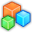# Microsoft Small BasicProgram Listing: WMX007
' Snake Game
' Copyright (C) 2009, Jason T. Jacques

' Game area and scaling controls
gameWidth = 32
gameHeight = 24
gridSize = 20

' Target frames per second
fps = 4

snakeColor = "#6495ED"
foodColor = "red"
pointsMultiply = 10

' Key controls
leftKey = "Left"
rightKey = "Right"

' Variable initialisation
snake = "snake"
initLength = 5
direction = 1 ' 1, North; 2, East; 3, South; 4, West

' Window title
snakeTitle = "Snake, Score: "

' Start game
Init()
Play()

' Initialise the world
Sub Init
GraphicsWindow.Hide()
GraphicsWindow.Title = snakeTitle + "0"
GraphicsWindow.CanResize = false
GraphicsWindow.Width = gameWidth * gridSize
GraphicsWindow.Height = gameHeight * gridSize

GraphicsWindow.BrushColor = snakeColor
Shapes.Move(Array.GetValue(snake, 1), (gameWidth / 2) * gridSize, (gameHeight / 2) * gridSize)
snakeLength = initLength

EndSub

Sub Play
GraphicsWindow.Show()

' Setup key events
GraphicsWindow.KeyDown = ChangeDirection

' Main loop
play = 1
While(play = 1)
Program.Delay(1000/fps)
MoveSnake()
CollisionCheck()
EndWhile

' Display end-game
EndGame()
EndSub

' Move the snake one grid unit in the current direction
Sub MoveSnake
' Move each segment one forward in the array
i = Array.GetItemCount(snake)
While (i > 0)
Array.SetValue(snake, i + 1, Array.GetValue(snake, i))
i = i - 1
EndWhile

' Create new snake segment and move to correct location
GraphicsWindow.BrushColor = snakeColor
If (direction = 1) Then
Shapes.Move(Array.GetValue(snake, 1), Shapes.GetLeft(Array.GetValue(snake, 2)), Shapes.GetTop(Array.GetValue(snake, 2)) - gridSize)
ElseIf(direction = 2) Then
Shapes.Move(Array.GetValue(snake, 1), Shapes.GetLeft(Array.GetValue(snake, 2)) + gridSize, Shapes.GetTop(Array.GetValue(snake, 2)))
ElseIf(direction = 3) Then
Shapes.Move(Array.GetValue(snake, 1), Shapes.GetLeft(Array.GetValue(snake, 2)), Shapes.GetTop(Array.GetValue(snake, 2)) + gridSize)
ElseIf(direction = 4) Then
Shapes.Move(Array.GetValue(snake, 1), Shapes.GetLeft(Array.GetValue(snake, 2)) - gridSize, Shapes.GetTop(Array.GetValue(snake, 2)))
EndIf

' Remove the tail end of the snake if we have reached the correct length
If(snakeLength < Array.GetItemCount(snake)) then
Shapes.Remove(Array.GetValue(snake, Array.GetItemCount(snake)))
Array.RemoveValue(snake, Array.GetItemCount(snake))
EndIf
EndSub

' Change heading of the snake
Sub ChangeDirection
If(GraphicsWindow.LastKey = rightKey) Then
direction = Math.Remainder(direction, 4) + 1
ElseIf(GraphicsWindow.LastKey = leftKey) Then
direction = Math.Remainder(direction + 2, 4) + 1
EndIf
EndSub

' Add a food item and store location data
GraphicsWindow.BrushColor = foodColor
foodX = (Math.GetRandomNumber(gameWidth) - 1) * gridSize
foodY = (Math.GetRandomNumber(gameHeight) - 1) * gridSize
Shapes.Move(food, foodX, foodY)
EndSub

' Remove food, increase snake length, add new food to world
Sub EatFood
' Remove food
Shapes.Remove(food)
' Increase snake length and update score
snakeLength = snakeLength + 1
GraphicsWindow.Title = snakeTitle + ((snakeLength - initLength) * pointsMultiply)
EndSub

' Check for collisions
Sub CollisionCheck
' Get position of head of snake

' Check if head is outside boundaries of world and end game
play = -1
ElseIf (headTop > (gameHeight - 1) * gridSize) Then
play = -2
play = -3
ElseIf (headLeft > (gameWidth - 1) * gridSize) Then
play = -4
EndIf

' Check if head has collided with other snake segment and end game
For i = 2 To Array.GetItemCount(snake)
play = 0
EndIf
EndIf
EndFor

' Check if head has collided with food and eat